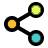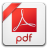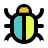Math Calculators, Lessons and Formulas

It is time to solve your math problem

mathportal.org

problem

Solve $\color{blue}{2x^2+3x-2 = 0}$ using the Quadratic Formula.

solution

The solutions are:

$$\color{blue}{ x_1 = -2 }~~ \text{and}~~ \color{blue}{ x_2 = \frac{ 1 }{ 2 } }$$

explanation

Step 1: Read the values of $a$, $b$, and $c$ from the quadratic equation: $a$ is the number in front of $x^2$, $b$ is the number in front of $x$, $c$ is the number at the end. In our case:

$$a = 2, \,\, b = 3, \,\, c = -2$$

Step 2: Plug in the values for $a$, $b$, and $c$ into the quadratic formula.

\begin{aligned} x_1,x_2 &= \frac{-b \pm \sqrt{b^2-4ac}}{2a} \\[1 em] x_1,x_2 &= \frac{ -3 \pm \sqrt{ 3 ^2 - 4 \cdot 2 \cdot (-2)} }{ 2 \cdot 2 } \end{aligned}

Step 3: Simplify expression under the square root.

$$x_1,x_2 = \frac{ -3 \pm \sqrt{ 25 } }{ 4 }$$

Step 4: Solve for $x$

\begin{aligned} & \color{blue}{ x_1 = \frac{ -3~-~\sqrt{ 25 } }{ 4 } = -2 } \\\\ & \color{blue}{ x_2 = \frac{ -3~+~\sqrt{ 25 } }{ 4 } = \frac{ 1 }{ 2 } } \end{aligned}

## Report an Error !

Form values: 2 , 1 , 3 , 2 , 2 , qf , g , Solve 2x^2+3x-2 = 0 by using quadratic formula - mathportal , Solve 2x^2+3x-2 = 0 using the Quadratic Formula.

Comment (optional)

## Share Result

Or just copy and paste the link wherever you need it.This calculator solves quadratic equations and provides an easy-to-understand step-by-step explanation. The solver uses three methods - completing the square, quadratic formula and factoring method.
show help ↓↓ examples ↓↓ tutorial ↓↓
$2x^2 + x - 3 = 0$
$\frac{1}{2}x^2+\frac{3}{4}x - 11 = 0$
$x^2+\sqrt{2}x - 4 = 0$
 Solve using factoring Solve by completing the square Solve by using quadratic formula
Allow the calculator to select the best method
working...
EXAMPLES
example 1:ex 1:
Solve for $x^2 + 3x - 4 = 0$ by factoring.
example 2:ex 2:
Solve $4x^2 - x - 3 = 0$ by completing the square.
example 3:ex 3:
Solve $-2x^2 - 0.5x + 0.75 = 0$ using the quadratic formula.
example 4:ex 4:
Solve $\frac{2}{3} x^2 - \frac{1}{3} x - 5 = 0$.
Search our database of more than 200 calculators
TUTORIAL

The most commonly used methods for solving quadratic equations are:

1. Factoring method

2. Completing the square

In the following sections, we'll go over these methods.

### Method 1A : Factoring method

If a quadratic trinomial can be factored, this is the best solving method.

We often use this method when the leading coefficient is equal to 1 or -1. If this is not the case, then it is better to use some other method.

Example 01: Solve $x^2 \color{red}{-8}x \color{blue}{+ 15} = 0$ by factoring.

Here we see that the leading coefficient is 1, so the factoring method is our first choice.

To factor this equation, we must find two numbers ( $a$ and $b$ ) with a sum is $a + b = \color{red}{8}$ and a product of $a \cdot b = \color{blue}{15}$.

After some trials and errors, we see that $a = 3$ and $b = 5$.

Now we use formula $x^2 - 8x + 15 = (x - a)(x - b)$ to get factored form:

$$x^2 - 8x + 15 = (x - 3)(x - 5)$$

Divide the factored form into two linear equations to get solutions.

\begin{aligned} x^2 - 8x + 15 &= 0 \\ (x - 3)(x - 5) &= 0 \\ x -3 &= 0 ~~ \text{or} ~~ x - 5= 0 \\ x &= 3 ~~ \text{or} ~~ x = 5 \end{aligned}

### Method 1B : Factoring - special cases

Example 02: Solve $x^2 -8x = 0$ by factoring.

In this case, (when the coefficient c = 0 ) we can factor out $\color{blue}{x}$ out of $x^2 - 8x$.

\begin{aligned} x^2 - 8x &= 0 \\ \color{blue}{x} \cdot ( x - 8 ) &= 0 \\ x &= 0 ~~ \text{or} ~~ x - 8 = 0 \\ x &= 0 ~~ \text{or} ~~ x = 8 \end{aligned}

Example 03: Solve $x^2 - 16 = 0$ by factoring.

In this case, ( when the middle term is equal 0) we can use the difference of squares formula.

\begin{aligned} x^2 - 16 &= 0 \\ x^2 - 4^2 &= 0 \text{ use } a^2 - b^2 = (a-b)(a+b) \\ (x - 4)(x+4) &= 0 \\ x - 4 &= 0 ~~ \text{or} ~~ x + 4 = 0 \\ x &= 4 ~~ \text{or} ~~ x = -4 \end{aligned}

### Method 3 : Solve using quadratic formula

This method solves all types of quadratic equations. It works best when solutions contain some radicals or complex numbers.

Example 05: Solve equation $2x^2 + 3x - 2 = 0$ by using quadratic formula.

Step 1: Read the values of $a$, $b$, and $c$ from the quadratic equation. ( $a$ is the number in front of $x^2$ , $b$ is the number in front of $x$ and $c$ is the number at the end)

$$a = 2 ~~ b = 3 ~~ \text{and} ~~ c = -2$$

Step 2:Plug the values for a, b, and c into the quadratic formula and simplify.

\begin{aligned} x_1, x_2 &= \frac{-b \pm \sqrt{b^2-4ac}}{2a} \\ x_1, x_2 &= \frac{-3 \pm \sqrt{3^2-4 \cdot 2 \cdot (-2) }}{2 \cdot 2} \\ x_1, x_2 &= \frac{-3 \pm \sqrt{9+ 16 }}{4} \\ x_1, x_2 &= \frac{-3 \pm \sqrt{25}}{4} \\ x_1, x_2 &= \frac{-3 \pm 5}{4} \end{aligned}

Step 3: Solve for $x_1$ and $x_2$

\begin{aligned} x_1 = & \frac{-3 \color{blue}{+} 5}{4} = \frac{2}{4} = \frac{1}{2} \\ x_2 = & \frac{-3 \color{blue}{-} 5}{4} = \frac{-8}{4} = -2 \end{aligned}

### Method 2 : Completing the square

This method can be used to solve all types of quadratic equations, although it can be complicated for some types of equations. The method involves seven steps.

Example 04: Solve equation $2x^2 + 8x - 10= 0$ by completing the square.

Step 1: Divide the equation by the number in front of the square term.

\begin{aligned} 2x^2 + 8x - 10 & = 0 ~~ / ~ \color{orangered}{:2} \\ \frac{2x^2}{2} + \frac{8x}{2} - \frac{10}{2} & = \frac{0}{2} \\ x^2 + 4x - 5 & = 0 \end{aligned}

Step 2: move $-5$ to the right:

$$x^2 + 4x = 5$$

Step 3: Take half of the x-term coefficient $\color{blue}{\dfrac{4}{2}}$, square it $\color{blue}{\left(\dfrac{4}{2} \right)^2}$ and add this value to both sides.

$$x^2 + 4x + \color{blue}{\left(\frac{4}{2} \right)^2} = 5 + \color{blue}{\left(\frac{4}{2} \right)^2}$$

Step 4: Simplify left and right side.

$$x^2 + 4x + 2^2 = 9$$

Step 5: Write the perfect square on the left.

$$\left( x + 2 \right)^2 = 9$$

Step 6: Take the square root of both sides.

\begin{aligned} x + 2 &= \pm \sqrt{9} \\\\ x + 2 &= \pm 3 \end{aligned}

Step 7: Solve for $x_1$ and $x_2$ .

\begin{aligned} x_1 & = +3 - 2 = 1 \\ x_2 & = -3 - 2 = - 5 \end{aligned}